1603033200

# A Complete Guide to Linear Regression for Beginners

What is supervised learning? In supervised learning, you have an input-output pair. And you will try to map the given input to output by training the input-output pair.

Another type of machine learning algorithm is unsupervised learning, in this, you don’t have an output variable. You will try to group the input variables by their similarities.

What is Regression? Regression is a statistical process of estimating the relationship between a dependant variable and several independent variables.

In other words, it can be said that linear regression suggests that the output variable can be represented as a linear combination of the input variables.

Linear Regression Example

Depending upon the number of input variables, linear regression can be classified into simple and multiple linear regression. If the number of input variables is one, then it is called simple linear regression.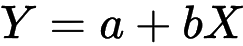Simple Linear Regression Formula

If there is more than one input variable, then it is called multiple linear regression.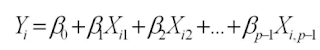Multiple Linear Regression Formula

In this blog post, I will be discussing simple linear regression.

## Buddha Community1598352300

## Regression: Linear Regression

Machine learning algorithms are not your regular algorithms that we may be used to because they are often described by a combination of some complex statistics and mathematics. Since it is very important to understand the background of any algorithm you want to implement, this could pose a challenge to people with a non-mathematical background as the maths can sap your motivation by slowing you down.In this article, we would be discussing linear and logistic regression and some regression techniques assuming we all have heard or even learnt about the Linear model in Mathematics class at high school. Hopefully, at the end of the article, the concept would be clearer.

**Regression Analysis **is a statistical process for estimating the relationships between the dependent variables (say Y) and one or more independent variables or predictors (X). It explains the changes in the dependent variables with respect to changes in select predictors. Some major uses for regression analysis are in determining the strength of predictors, forecasting an effect, and trend forecasting. It finds the significant relationship between variables and the impact of predictors on dependent variables. In regression, we fit a curve/line (regression/best fit line) to the data points, such that the differences between the distances of data points from the curve/line are minimized.

#regression #machine-learning #beginner #logistic-regression #linear-regression #deep learning1603033200

## A Complete Guide to Linear Regression for Beginners

What is supervised learning? In supervised learning, you have an input-output pair. And you will try to map the given input to output by training the input-output pair.

Another type of machine learning algorithm is unsupervised learning, in this, you don’t have an output variable. You will try to group the input variables by their similarities.

What is Regression? Regression is a statistical process of estimating the relationship between a dependant variable and several independent variables.

In other words, it can be said that linear regression suggests that the output variable can be represented as a linear combination of the input variables.

Linear Regression Example

Depending upon the number of input variables, linear regression can be classified into simple and multiple linear regression. If the number of input variables is one, then it is called simple linear regression.Simple Linear Regression Formula

If there is more than one input variable, then it is called multiple linear regression.Multiple Linear Regression Formula

In this blog post, I will be discussing simple linear regression.1594271340

## A Deep Dive into Linear Regression

Let’s begin our journey with the truth — machines never learn. What a typical machine learning algorithm does is find a mathematical equation that, when applied to a given set of training data, produces a prediction that is very close to the actual output.

Why is this not learning? Because if you change the training data or environment even slightly, the algorithm will go haywire! Not how learning works in humans. If you learned to play a video game by looking straight at the screen, you would still be a good player if the screen is slightly tilted by someone, which would not be the case in ML algorithms.

However, most of the algorithms are so complex and intimidating that it gives our mere human intelligence the feel of actual learning, effectively hiding the underlying math within. There goes a dictum that if you can implement the algorithm, you know the algorithm. This saying is lost in the dense jungle of libraries and inbuilt modules which programming languages provide, reducing us to regular programmers calling an API and strengthening further this notion of a black box. Our quest will be to unravel the mysteries of this so-called ‘black box’ which magically produces accurate predictions, detects objects, diagnoses diseases and claims to surpass human intelligence one day.

We will start with one of the not-so-complex and easy to visualize algorithm in the ML paradigm — Linear Regression. The article is divided into the following sections:

1. Need for Linear Regression

2. Visualizing Linear Regression

3. Deriving the formula for weight matrix W

4. Using the formula and performing linear regression on a real world data set

Note: Knowledge on Linear Algebra, a little bit of Calculus and Matrices are a prerequisite to understanding this article

Also, a basic understanding of python, NumPy, and Matplotlib are a must.

## 1) Need for Linear regression

Regression means predicting a real valued number from a given set of input variables. Eg. Predicting temperature based on month of the year, humidity, altitude above sea level, etc. Linear Regression would therefore mean predicting a real valued number that follows a linear trend. Linear regression is the first line of attack to discover correlations in our data.

Now, the first thing that comes to our mind when we hear the word linear is, a line.

Yes! In linear regression, we try to fit a line that best generalizes all the data points in the data set. By generalizing, we mean we try to fit a line that passes very close to all the data points.

But how do we ensure that this happens? To understand this, let’s visualize a 1-D Linear Regression. This is also called as Simple Linear Regression

#calculus #machine-learning #linear-regression-math #linear-regression #linear-regression-python #python1592023980

## 5 Regression algorithms: Explanation & Implementation in Python

Take your current understanding and skills on machine learning algorithms to the next level with this article. What is regression analysis in simple words? How is it applied in practice for real-world problems? And what is the possible snippet of codes in Python you can use for implementation regression algorithms for various objectives? Let’s forget about boring learning stuff and talk about science and the way it works.

#linear-regression-python #linear-regression #multivariate-regression #regression #python-programming1595989620

## Linear Regression : Beginner’s Approach

Before Starting the blog lets us refresh some of the basic definitions

# What is machine Learning?

Machine learning (ML) is the study of computer algorithms that improve automatically through experience.It is seen as a subset of artificial intelligence. Machine learning algorithms build a mathematical model based on sample data, known as “training data”, in order to make predictions or decisions without being explicitly programmed to do so .Machine learning algorithms are used in a wide variety of applications, such as email filtering and computer vision.

source: Wikipedia

As, per the above definition the models require training data to compute predictions. Depending upon the data provided the machine learning algorithm can be broadly classified into 3 categories namely supervised, unsupervised and semi supervised learning.

In this blog, we are going to learn about one such supervised learning algorithm.

Supervised Algorithms basically can be divided into two categories:

## Classification:

In this type of problem the machine learning algorithm will specify the data belongs to which set of class. The classes can be binary as well as multiple class.

E.g. Logistic Regression, Decision Tree, Random Forest, Naïve Bayes.

## Regression:

In this type of problems the machine learning algorithm will try to find the relationship between continuous of variables. The modelling approach used here is between a dependent variable (response variable) with a given set of independent (feature) variables. Just fyi I will be using Response and target variable’s interchangeably along with LR for Linear Regression.

E.g. Linear Regression, Ridge Regression, Elastic Net Regression.

Today we are going to learn Simple Linear Regression.

## Linear Regression:

I personally believe to learn any machine learning model or any concept related to it if we understand the geometric intuition behind it then it will stay longer with us as visuals helps sustain memory as compared to a mathematical representation.

So, to proceed with the blog we will be categorizing into below aspects for LR:

1. What is Linear Regression?

2. Geometric Intuition

3. Optimization Problem

4. Implementation with sklearn Library.

## What is Linear Regression?

As stated, above LR is a type of Regression Technique which tries to find relation between continuous set of variables from any given dataset.

So, the problem statement that algorithm tries to solve linearly is to best fit a line/plane/hyperplane (as the dimension goes on increasing) for any given set of data. Yes, It’s that simple

We understand this with the help of below scatter Plot.

## Geometric Intuition:

To represent visually let’s take a look at below scatter plot which is derived from Boston Housing Data set which I have used as an example in the latter part of the blog.

The co-ordinates are denoted as below,

X-Axis -> Actual House Prices

Y-Axis -> Predicted house Prices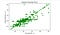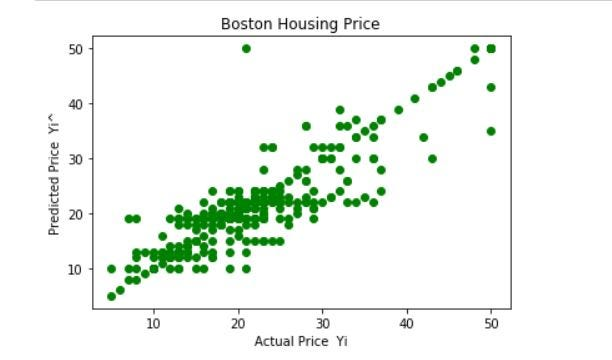Figure -1

People I want you to stay with me on this as we need to try to understand what LR is doing visually So, having a look at the scatter plot if we visualize and try to pass an imaginary line from the origin then the line will be passing from most the predicted points which shows that model has correctly plotted most of the response variables.

## Optimization Problem:

Now, as we are getting hold of what LR does visually let’s have a look at the optimization problem that we are going to solve because every machine learning algorithm boil’s down to an optimization problem where we can understand the crux of it through a mathematical equation.

For any Machine learning algo the ultimate goal is to reduce the error’s in the dataset so that it can predict the target variable’s more accurately.

With that said what is the optimization problem for Linear Regression? Which is used to minimize sum of errors across the Training data. The next question which will pop up in your mind is why sum of errors?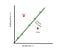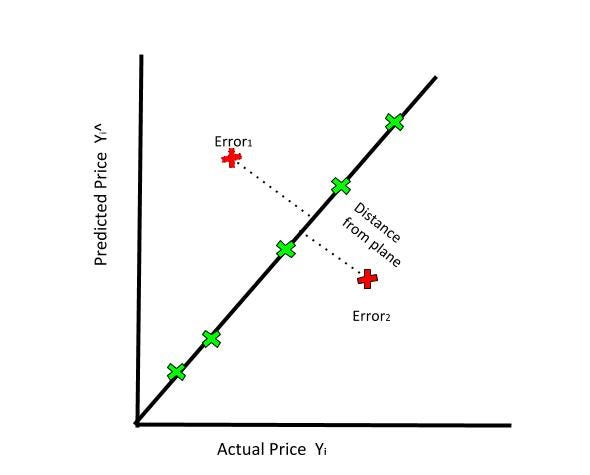The above image which is a lighter version of the scatter plot answers that question perfectly, so as you can see we have drawn a line passing through the origin which will have the points which are correctly classified denoted by green cross. But also, if we look closely there are few of the points which are not present on that line denoted by red cross. These points can be differentiated as points above and below that line. These points can be defined as data point which the model was not able to predict it correctly. And the optimization problem is used to reduce the distance of these error points.

#linear-regression #beginners-guide #machine-learning #deep learning